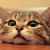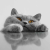## 计算：有10个小孩围 成一圈并依次编号，教师指定从第2个小孩开始报数，报到第3个小孩即令其出列。然后从下一个孩子继续报数，数到第3个小孩又令其出列，如此直到所有的孩子都出列。求小孩出列的先后顺序。

00
```<?php
\$arr = array(1,2,3,4,5,6,7,8,9,10);
function reOrder(\$start=0,\$arr=array()){
\$new_arr = array();
\$s = \$start-1;
for(\$i=1 ; \$i<=count(\$arr) ; \$i++){
for(\$j=0 ; \$j<3 ; \$j++){
if(\$s >= count(\$arr))\$s=0;
if(\$arr[\$s]!='none'){
if(\$j==2){
\$new_arr[] = \$arr[\$s];
\$arr[\$s] = 'none';
}
}else{
\$j--;
}
\$s++;
}
}
return \$new_arr;
}
print_r(reOrder(2,\$arr));
?>```

。。。那要什么样的呢。。。 文字版？
0```public static void main(String[] args) {
List<Integer> list = new ArrayList<Integer>();
List<Integer> remove = new ArrayList<Integer>();
int start = 2;
int key = 3;
list.add(1);
list.add(2);
list.add(3);
list.add(4);
list.add(5);
list.add(6);
list.add(7);
list.add(8);
list.add(9);
list.add(10);
list.add(11);
list.add(12);

remove = getRemoveList(list, remove, start + key-1, key);

for (int i = 0; i < remove.size(); i++) {
System.out.print(remove.get(i)+",");
}
}

public static List<Integer> getRemoveList(List<Integer> list,
List<Integer> remove, int start, int key) {
if (list.size() == 1) {
remove.add(list.get(0));
return remove;
} else {
int i = 0;
while (i * key + start - 1 - i < list.size()) {
remove.add(list.get(i * key + start - 1 - i));
list.remove(i * key + start - 1 - i);
i++;
}

return getRemoveList(list, remove, key - (i * key + start) % key-1,key);
}
}```